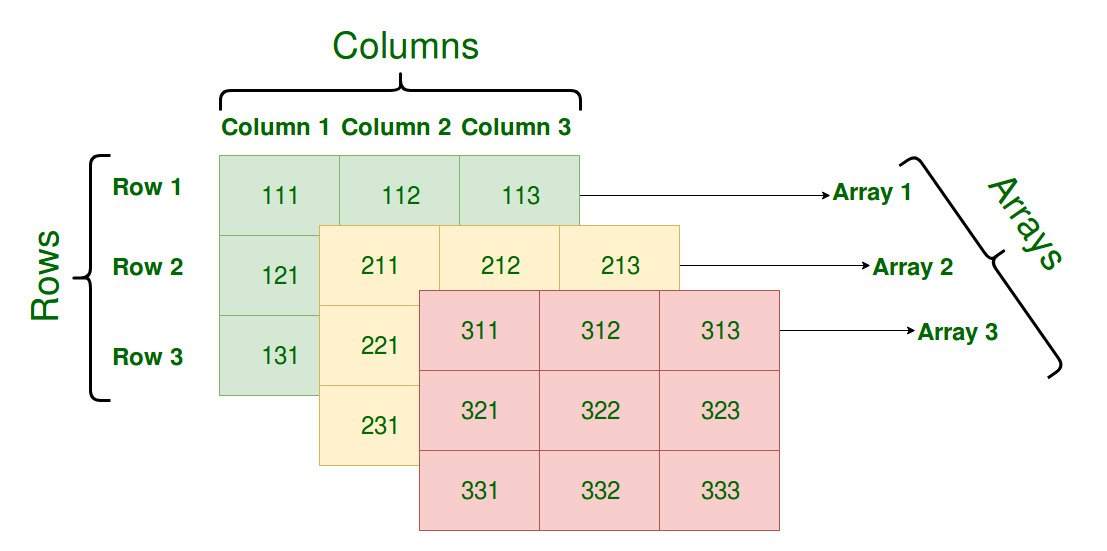Related Articles

# Multidimensional Arrays in C / C++

• Difficulty Level : Easy
• Last Updated : 17 Feb, 2021

Array- Basics
In C/C++, we can define multidimensional arrays in simple words as array of arrays. Data in multidimensional arrays are stored in tabular form (in row major order).

General form of declaring N-dimensional arrays:

```data_type  array_name[size1][size2]....[sizeN];

data_type: Type of data to be stored in the array.
Here data_type is valid C/C++ data type
array_name: Name of the array
size1, size2,... ,sizeN: Sizes of the dimensions
```

Examples:

```Two dimensional array:
int two_d;

Three dimensional array:
int three_d;
```

Size of multidimensional arrays

Total number of elements that can be stored in a multidimensional array can be calculated by multiplying the size of all the dimensions.
For example:
The array int x can store total (10*20) = 200 elements.
Similarly array int x can store total (5*10*20) = 1000 elements.

### Two-Dimensional Array

Two – dimensional array is the simplest form of a multidimensional array. We can see a two – dimensional array as an array of one – dimensional array for easier understanding.

• The basic form of declaring a two-dimensional array of size x, y:
Syntax:
```data_type array_name[x][y];
data_type: Type of data to be stored. Valid C/C++ data type.
```
• We can declare a two dimensional integer array say ‘x’ of size 10,20 as:
```int x;
```
• Elements in two-dimensional arrays are commonly referred by x[i][j] where i is the row number and ‘j’ is the column number.
• A two – dimensional array can be seen as a table with ‘x’ rows and ‘y’ columns where the row number ranges from 0 to (x-1) and column number ranges from 0 to (y-1). A two – dimensional array ‘x’ with 3 rows and 3 columns is shown below:Initializing Two – Dimensional Arrays: There are two ways in which a Two-Dimensional array can be initialized.
First Method:

```int x = {0, 1 ,2 ,3 ,4 , 5 , 6 , 7 , 8 , 9 , 10 , 11}
```

The above array have 3 rows and 4 columns. The elements in the braces from left to right are stored in the table also from left to right. The elements will be filled in the array in the order, first 4 elements from the left in first row, next 4 elements in second row and so on.

Better Method:

```int x = {{0,1,2,3}, {4,5,6,7}, {8,9,10,11}};
```

This type of initialization make use of nested braces. Each set of inner braces represents one row. In the above example there are total three rows so there are three sets of inner braces.

Accessing Elements of Two-Dimensional Arrays: Elements in Two-Dimensional arrays are accessed using the row indexes and column indexes.
Example:

```int x;
```

The above example represents the element present in third row and second column.

Note: In arrays if size of array is N. Its index will be from 0 to N-1. Therefore, for row index 2 row number is 2+1 = 3.

To output all the elements of a Two-Dimensional array we can use nested for loops. We will require two for loops. One to traverse the rows and another to traverse columns.

 `// C++ Program to print the elements of a``// Two-Dimensional array``#include``using` `namespace` `std;`` ` `int` `main()``{``    ``// an array with 3 rows and 2 columns.``    ``int` `x = {{0,1}, {2,3}, {4,5}};`` ` `    ``// output each array element's value``    ``for` `(``int` `i = 0; i < 3; i++)``    ``{``        ``for` `(``int` `j = 0; j < 2; j++)``        ``{``            ``cout << ``"Element at x["` `<< i``                 ``<< ``"]["` `<< j << ``"]: "``;``            ``cout << x[i][j]<

Output:

```Element at x: 0
Element at x: 1
Element at x: 2
Element at x: 3
Element at x: 4
Element at x: 5
```

### Three-Dimensional ArrayInitializing Three-Dimensional Array: Initialization in Three-Dimensional array is same as that of Two-dimensional arrays. The difference is as the number of dimension increases so the number of nested braces will also increase.
Method 1:

```int x = {0, 1, 2, 3, 4, 5, 6, 7, 8, 9, 10,
11, 12, 13, 14, 15, 16, 17, 18, 19,
20, 21, 22, 23};
```

Better Method:

```int x =
{
{ {0,1,2,3}, {4,5,6,7}, {8,9,10,11} },
{ {12,13,14,15}, {16,17,18,19}, {20,21,22,23} }
};
```

Accessing elements in Three-Dimensional Arrays: Accessing elements in Three-Dimensional Arrays is also similar to that of Two-Dimensional Arrays. The difference is we have to use three loops instead of two loops for one additional dimension in Three-dimensional Arrays.

 `// C++ program to print elements of Three-Dimensional``// Array``#include``using` `namespace` `std;`` ` `int` `main()``{``    ``// initializing the 3-dimensional array``    ``int` `x =``    ``{``        ``{ {0,1}, {2,3}, {4,5} },``        ``{ {6,7}, {8,9}, {10,11} }``    ``};`` ` `    ``// output each element's value``    ``for` `(``int` `i = 0; i < 2; ++i)``    ``{``        ``for` `(``int` `j = 0; j < 3; ++j)``        ``{``            ``for` `(``int` `k = 0; k < 2; ++k)``            ``{``                ``cout << ``"Element at x["` `<< i << ``"]["` `<< j``                     ``<< ``"]["` `<< k << ``"] = "` `<< x[i][j][k]``                     ``<< endl;``            ``}``        ``}``    ``}``    ``return` `0;``}`

Output:

```Element at x = 0
Element at x = 1
Element at x = 2
Element at x = 3
Element at x = 4
Element at x = 5
Element at x = 6
Element at x = 7
Element at x = 8
Element at x = 9
Element at x = 10
Element at x = 11
```

In similar ways, we can create arrays with any number of dimension. However the complexity also increases as the number of dimension increases.
The most used multidimensional array is the Two-Dimensional Array.

This article is contributed by Harsh Agarwal. If you like GeeksforGeeks and would like to contribute, you can also write an article using contribute.geeksforgeeks.org or mail your article to contribute@geeksforgeeks.org. See your article appearing on the GeeksforGeeks main page and help other Geeks.